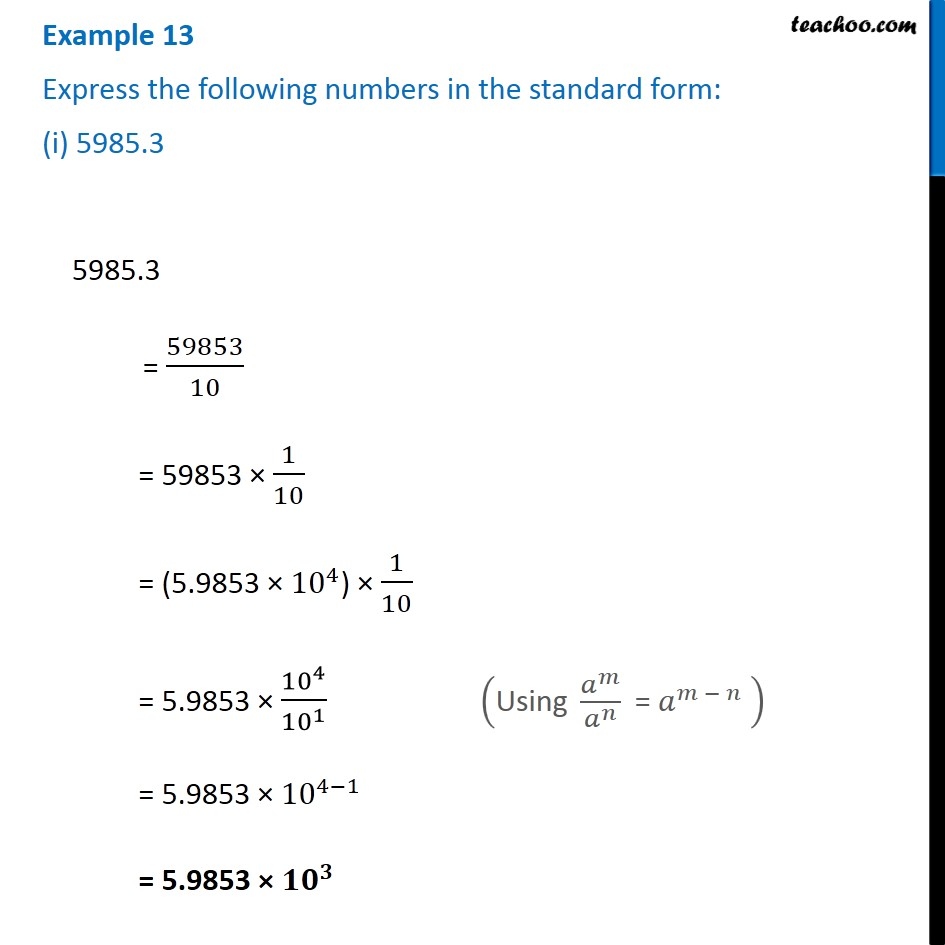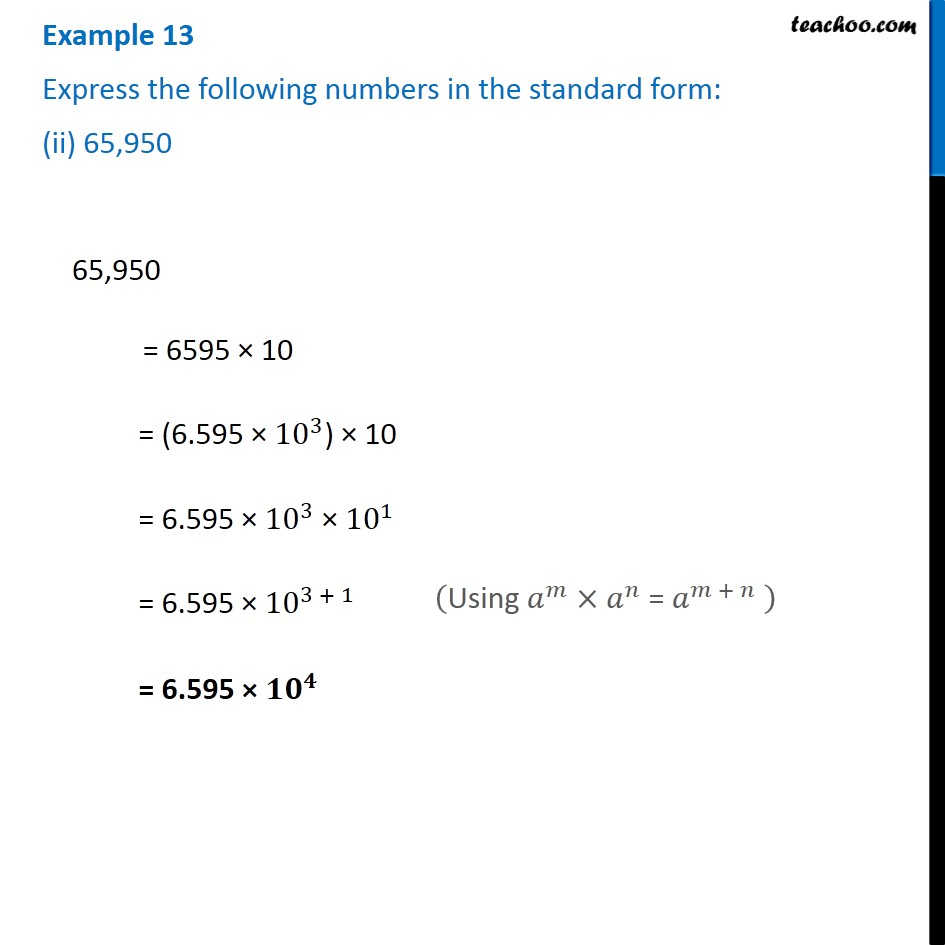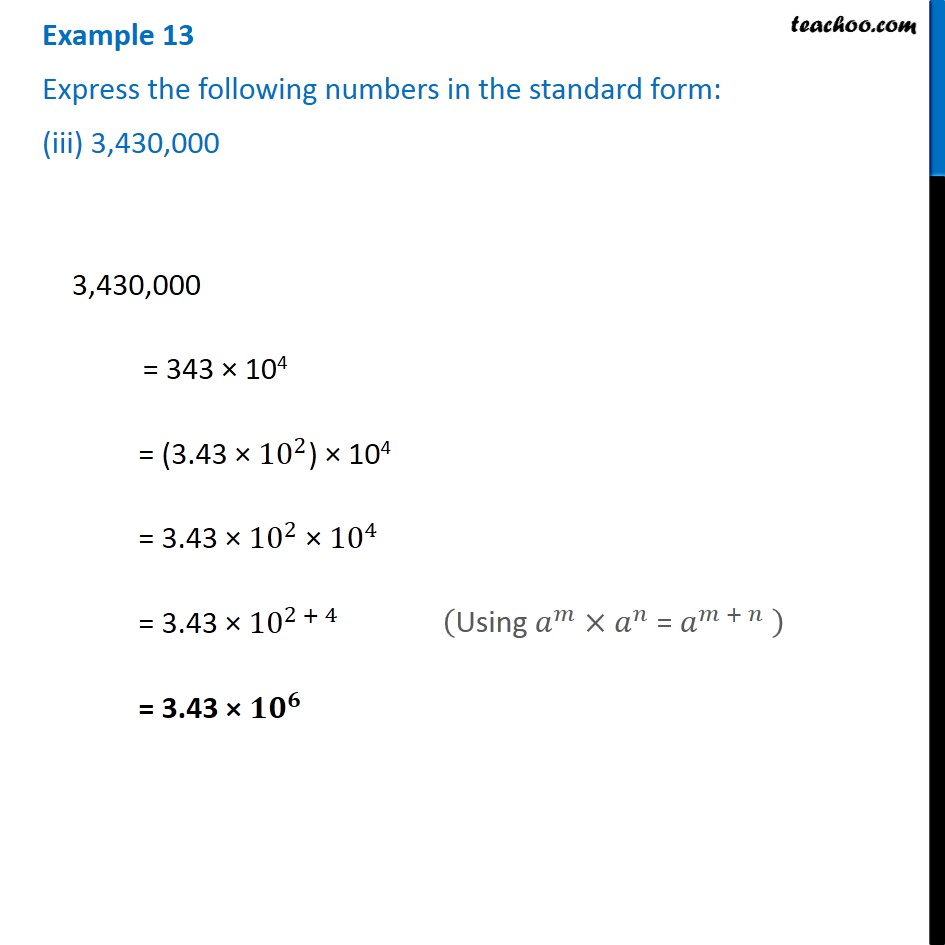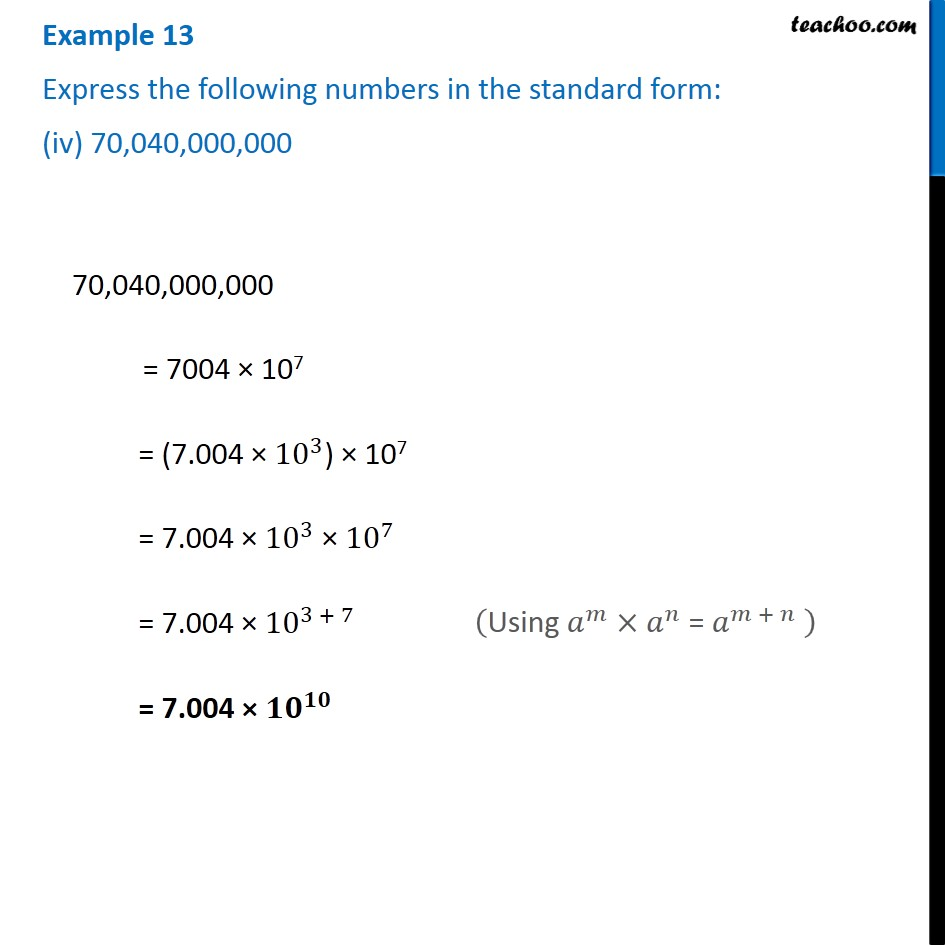1. Chapter 13 Class 7 Exponents and Powers
2. Concept wise
3. Standard Form

Transcript

Example 13 Express the following numbers in the standard form: (ii) 65,950 ("Using " 𝑎^𝑚×𝑎^𝑛 " = " 𝑎^(𝑚 + 𝑛) " " ) Example 13 Express the following numbers in the standard form: (iii) 3,430,000 3,430,000 = 343 × 104 = (3.43 × 〖10〗^2) × 104 = 3.43 × 〖10〗^2 × 〖10〗^4 = 3.43 × 〖10〗^(2 + 4) = 3.43 × 〖𝟏𝟎〗^𝟔 ("Using " 𝑎^𝑚×𝑎^𝑛 " = " 𝑎^(𝑚 + 𝑛) " " ) Example 13 Express the following numbers in the standard form: (iv) 70,040,000,000 70,040,000,000 = 7004 × 107 = (7.004 × 〖10〗^3) × 107 = 7.004 × 〖10〗^3 × 〖10〗^7 = 7.004 × 〖10〗^(3 + 7) = 7.004 × 〖𝟏𝟎〗^𝟏𝟎

Standard Form